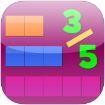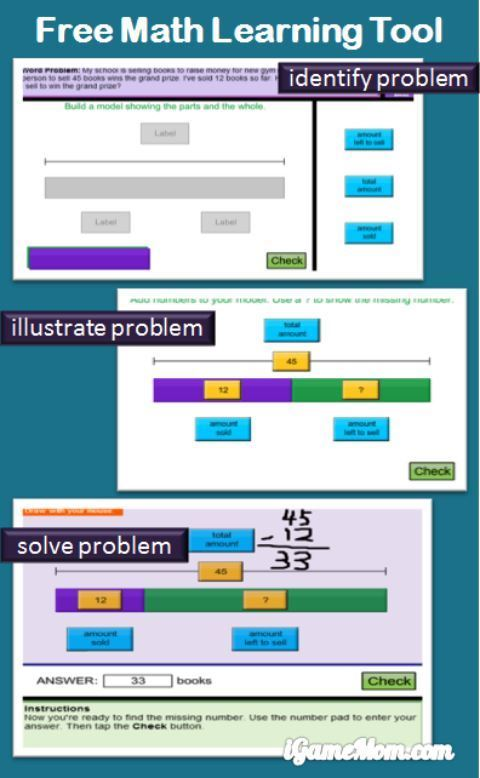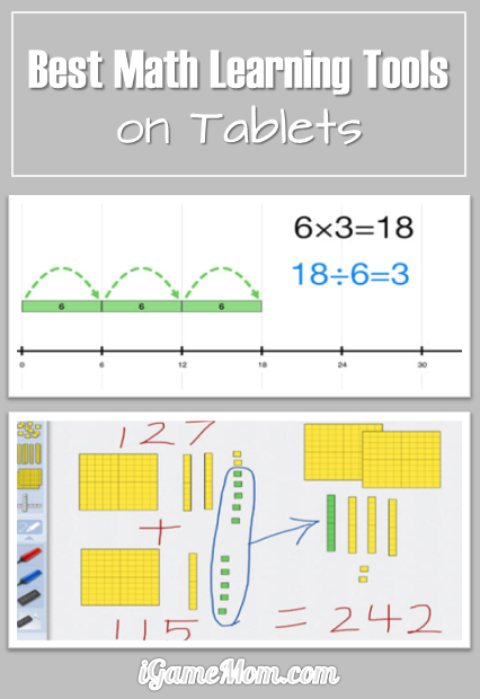# Free App: Math Learning Tool Helping Kids Think Through Math ProblemsI always believe math is a tool for kids to learn how to think, besides doing number calculations. The skill of thinking includes asking the right questions and exploring possible solutions. That is why I like Singapore math. I especially like the model drawing process that helping kids learn how to think when they are asked a number question. I am extremely happy to find 4 free apps that using Singapore math model method to teach kids math. They are also available online as a web math learning tool for free.To really explain how the model method works, we need use an example. Here is a math problem: students are selling books to raise money for school. The first person to sell 45 books wins the grand prize. A child sold 12 books so far. How many more books must he sell to win the grand prize?

To solve the problem, kids will draw a long bar representing the total number of books they need sell to win the prize, and mark a portion of it as the number of books the kid already sold. They then label each part of the bar with numbers they know, and figure out the part of the bar that represent the number of additional books the kid need sell to win the prize. In the graph above, it is the green part of the bar.

With the help of the model, kids can easily see it is a subtraction problem, they need use 45 minus 12 to find out the answer to the problem.

At the bottom of the screen there is always a note telling kids what they need do for the next step. Kids also have drawing pad to do some calculation on the screen if they need to.

There are 4 apps in the series, one for addition and subtraction, one for multiplication and division, one for ratio, and one for fraction. All 4 apps are free. All 4 are available on iPad. If you don’t have iPads, you can use the web version on computer. This is the web address: Think Blocks . Below the app links are in order of Addition and Subtraction, Multiplication and Division, Fraction, and Ratio.

If you would like to check it out or purchase it, please use the App Store link provided below. The cost is the same to you, but iGameMom gets a small percentage. Thanks for your support! Note: The link works for all countries.Looking for more Math Learning Tools? Check out Best Math Learning Tools on iPad and Other Tablets.